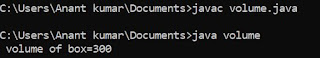## Friday, 27 July 2018

### What is strong number ?

A Number is called strong number if the sum of factorial of it digits is equal to itself.

Example :  145

### Program to check strong number ::

#include <stdio.h>
void main ()
{
int n , add = 0 , a , i , temp ;
clrscr () ;
printf ("\n Enter a number:") ;
scanf ("%d", &n) ;
temp = n ;
while ( n )
{
i = 1 ;
fact = 1 ;
a = n % 10 ;
while ( i <= a )
{
fact = fact * i ;
i++ ;
}
n = n / 10 ;
}
if (  add = temp )
{
printf ("\n it is strong number:") ;
else
printf ("\n it is not strong number:") ;
getch() ;
}

This is a program to check a given number is strong number or not . Here we have to input a number from the user and then apply condition for strong number.

Output screen ::

Enter a number :: 45
It is not strong number
Enter a number :: 145
It is strong number